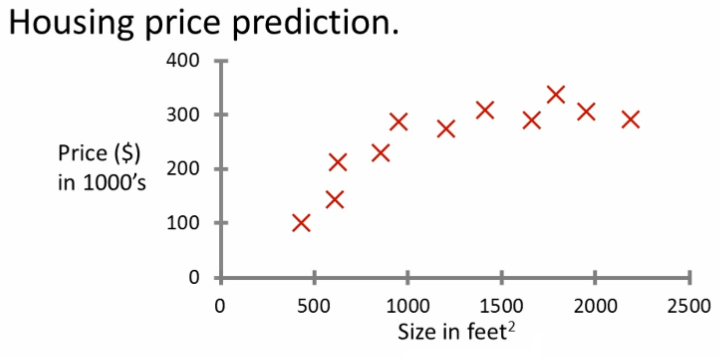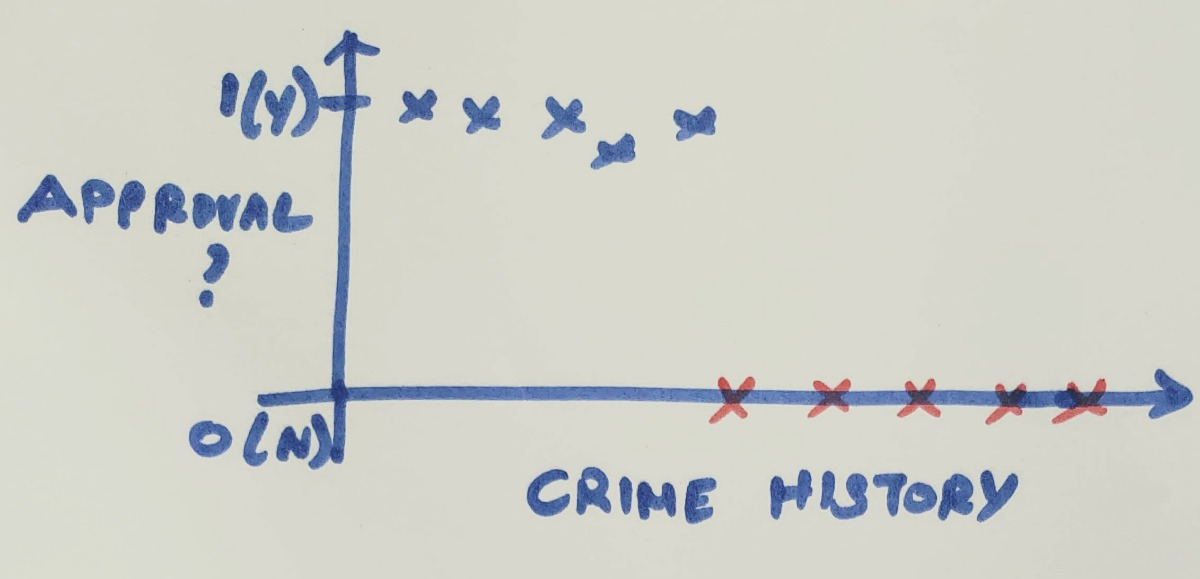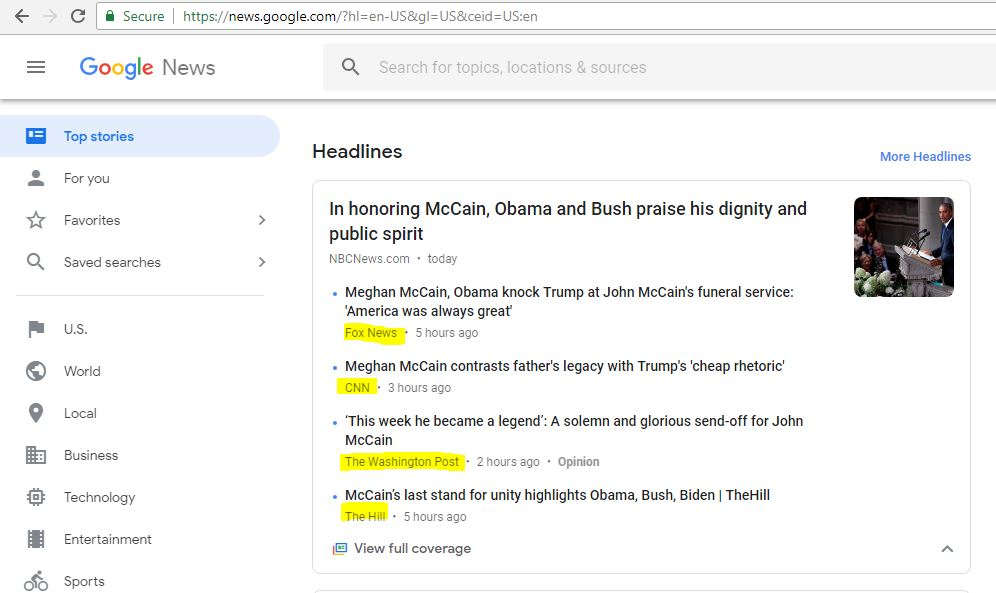02 Sep 18 · npack · #ml ·   Bookmark   ×

# Types of Machine learning algorithms (Part 2 of 6)

Now that we have completed the fundamentals of machine learning, lets dive in to the types of machine learning algorithms. There are three types of machine learning,

• Supervised learning
• Unsupervised learning
• Reinforcement learning

## Supervised learning

Its easier to grasp the concepts using an example: Lets consider the classic example from Andrew-ag's course.  We have a data set with the house sq.ft and the prices they sold for. We can use this data to create a 2d plot like the below diagram and train our model.

When we get a new query, say for example your friend wants to sell a 750sq.ft house, you can plot it in the model and we can predict how much it would sell for (approx 150k \$)We call the above method as supervised learning. As the name implies, we train the model in this algorithm with a training data set with the correct answers, the algorithm learns the patterns from the training data. Learning stops when the algorithm achieves an acceptable level of performance. The majority of practical machine learning uses supervised learning.

There are two types of Supervised learning algorithms:

• Regression : Predict a continuous valued output. Example: predicting house prices
• Classification: Predict discrete values. Example: Predict whether its a dog or cat

Here's an example for classification problem:

We have access to crime records of the applicants and we have to classify the applicants as approved or rejected. With more crime, the applicants get rejected. Those with minor violations are approved. So here we are predicting discrete (Approved / Rejected) values, so this is a Classification problem## Unsupervised learning:

In Unsupervised learning, there is no training dataset with the correct answers. Instead we let the system load all the data and find structure / patterns in the data on its own. So essentially the machine eats all the data, visualizes it and find groups / clusters in the data

For example:  when you visit news.google.com , All the news articles from different sources (news outlets) are being grouped into one based on the similarity, that is an example for clustering problem.More examples include, social network analysis,  classifying a customer into a segment etc...

Unsupervised learning problems can be further grouped into clustering and association problems.

• Clustering: A clustering problem is where you want to discover the inherent groupings in the data, such as grouping customers by purchasing behavior.
• Association: An association rule learning problem is where you want to discover rules that describe large portions of your data, such as people that buy X also tend to buy Y

## Reinforcement learning:

Reinforcement learning is an important type of Machine Learning where an agent learns on its own how to behave in a environment by performing actions and seeing the results. In other words, the machine takes an action, learns from its failures and auto corrects itself next time.

The idea behind Reinforcement Learning is that an agent will learn from the environment by interacting with it and receiving rewards for performing actions.

For the scope of this training we will not get much deep into this part